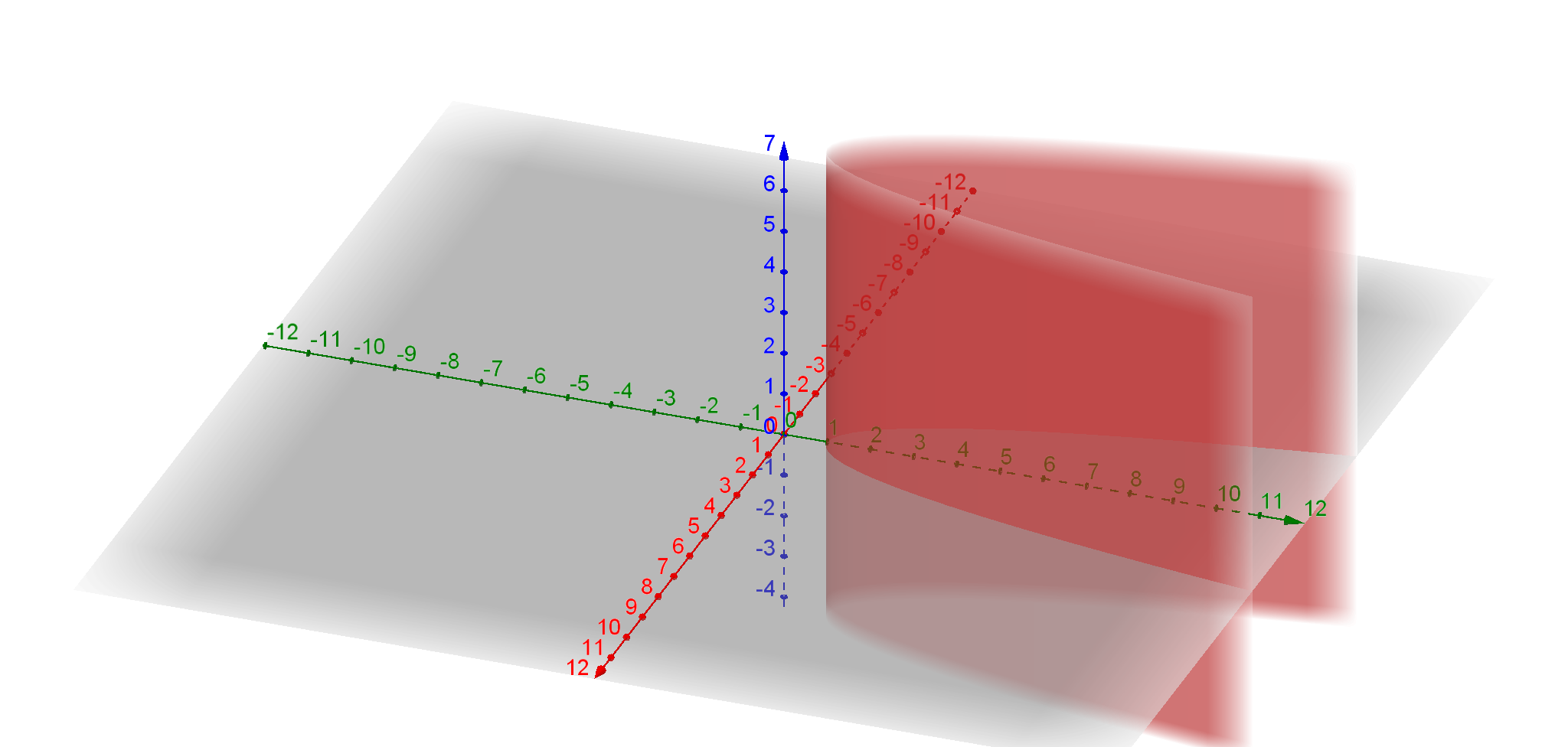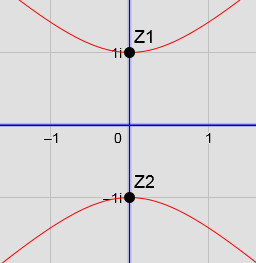# Imaginary Number Roots Intersecting With Z Axis

Dave Andrews shared this question 2 years ago

I am teaching myself and only have high school math in terms of formal training.(62 Yrs)

I dont really know how this site works but here goes.

Per the attached -I would expect to see intersection on the Z axis at + and - i for y= x^2 +1

Removing the x and y axis does not remove the element of dimension either .ie just plotting on z axis only

What's a tag ? referred to (in context of) below ?Hi,

The expression y = x^2 + 1, for complex numbers gives you two solutions: i and -i, the imaginary constant i = (-1)^(1/2)

In the complex plane the above expression can be written as w = z^2+1, which cannot be represented easily since it lives in the 4 dimensional space. Instead, we need to use some techniques to be able to see it. If you plot the real and imaginary part of this expression separately then we can get a picture of the two roots: i, and -i. The image you should get is the following:It was made with this applet: https://www.geogebra.org/m/ZPzSWzX7

If you are learning complex analysis the videos recommender by @mathmagic are great. I would like to recommend also this online book:

Kind regards.1

What do you want exactly? That surface doesn't cross the z-axis (blue)1

Thanks Michael. Where would the two roots (+) and (- ) i reflect ?1

Nothing for the graph I think. If you set y=0 then

x^2+1 = 0

x^2 = -1

x^2 = i or -i1

Thanks,subsequent comments helped me with this.1

este es el capitulo 13 de algo que te puede resultar interesante desde el capitulo 11

Hi,

The expression y = x^2 + 1, for complex numbers gives you two solutions: i and -i, the imaginary constant i = (-1)^(1/2)

In the complex plane the above expression can be written as w = z^2+1, which cannot be represented easily since it lives in the 4 dimensional space. Instead, we need to use some techniques to be able to see it. If you plot the real and imaginary part of this expression separately then we can get a picture of the two roots: i, and -i. The image you should get is the following:It was made with this applet: https://www.geogebra.org/m/ZPzSWzX7

If you are learning complex analysis the videos recommender by @mathmagic are great. I would like to recommend also this online book:

Kind regards.1

Thank you1

hola

me permito sugerirte añadir un par de lineas a la ventana algebraica

0=e

y

0=f

no sé si valdrá en general pero creo que setía util1

Te refieres a este applet https://www.geogebra.org/m/ZPzSWzX7?

Por cierto, tengo la mala suerte que las notificaciones de este forum me llegan de forma aleatoria, a veces sí, a veces no. Hoy he entrado aquí para ver si había más comentarios.

Saludos1

Hi, there was a problem with this applet so I had to reupload another version.

Cheers1

era solo una sugerencia para que se viera la proyección1

Oh, ya entendi. Gracias. Definitivamente la agreagre, nada mas que me deje el servidor :)1

Oh si que me gusta. Creo que lo habia intentado cuando hice este applet pero no sabia como definir la curva de nivel. Ahora se ve bastante bien. Gracias de nuevo.1

el problema que tuviste es muy simple y por lo tanto complicadísimo de ver ; yo todavía caigo y me encorajino

si escribes f=0 redefines f ; si escribes 0=f creas una curva implícita